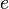# Orbital eccentricity facts for kids

Kids Encyclopedia Facts
(Redirected from Eccentricity (orbit))

In astrodynamics, orbital eccentricity shows how much the shape of an object's orbit is different from a circle.

Eccentricity ($e\,\!$) is defined for all circular, elliptic, parabolic and hyperbolic orbits. It can take the following values:

• for circular orbits:$e\,\!$ is equal to zero,
• for elliptic orbits:$e\,\!$ is more than zero but less than 1,
• for parabolic trajectories:$e\,\!$ is equal to 1,
• for hyperbolic trajectories:$e\,\!$ is more than 1.

## Finding eccentricity

Use this formula:$e_{obj}=\frac {r_a-r_p} {r_a+r_p}$, where eobj is the eccentricity, ra is the apoapsis (far point) of the object's orbit, and rp is the periapsis (near point) of the object's orbit. The near and far points are the apsides.

## Images for kids

ru:Кеплеровы элементы орбиты#ЭксцентриситетOrbital eccentricity Facts for Kids. Kiddle Encyclopedia.